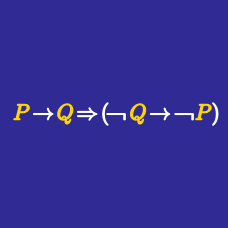Logic

# Logic Gates Intermediate Warmup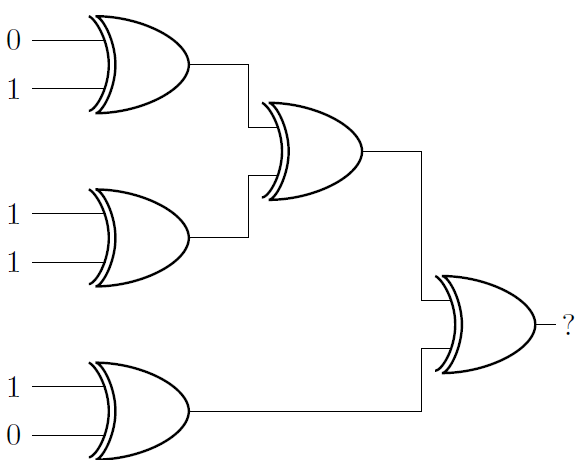The image above has five XOR gates. XOR gates output a 1 if the inputs are a 1 and a 0, otherwise it outputs a 0.

What's the output at the end?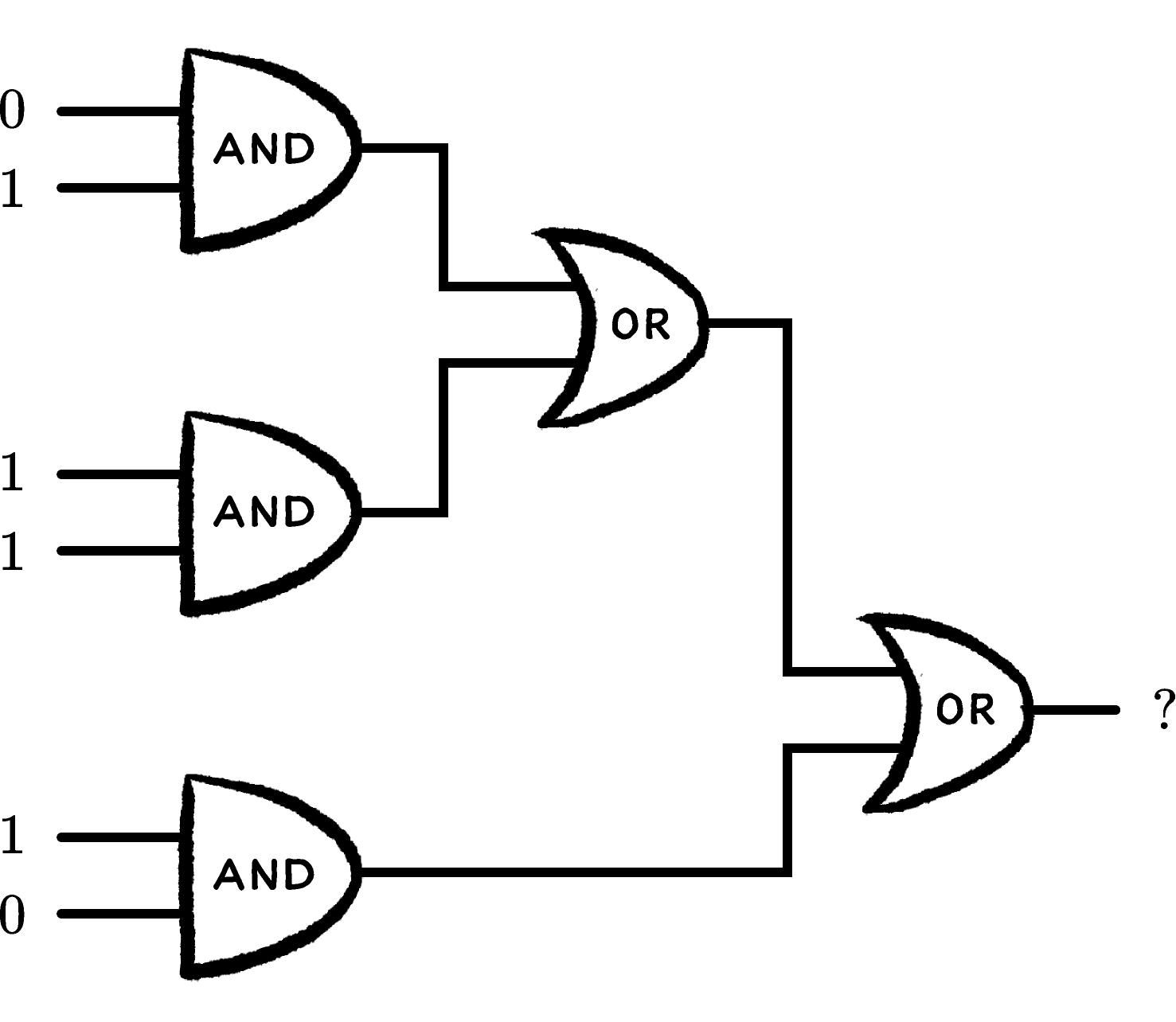In the image above there are three AND gates and two OR gates. What's the question mark?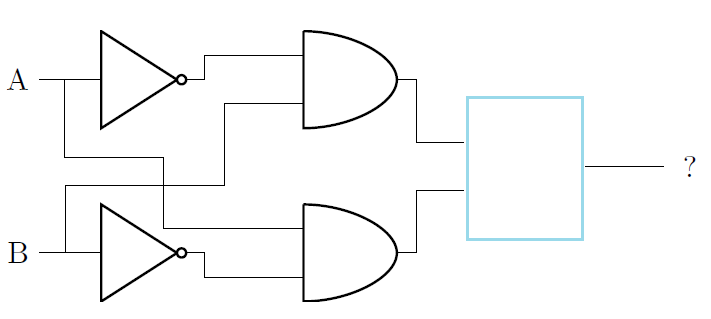The setup above works like a XOR gate (the output will be 1 if exactly one of the inputs is 1). However, the gate marked by a blue box is missing. What is the gate?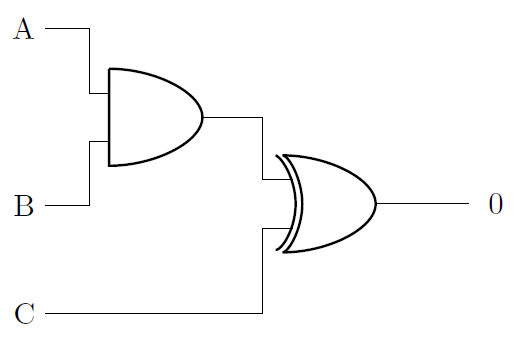If A, B, and C are assigned values of 0 or 1 randomly and independently, what is the probability that the above diagram will be correct?

HINT: Of the 8 possible sets of inputs, 4 involve $C = 1$ and 4 involve $C = 0 .$ Treat them separately.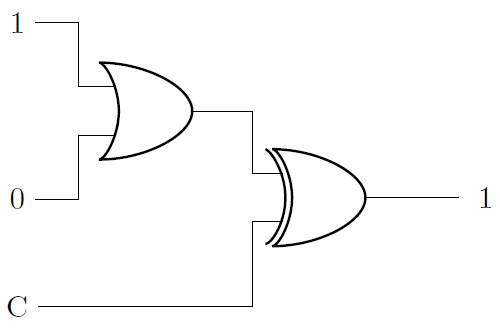What is C?

×